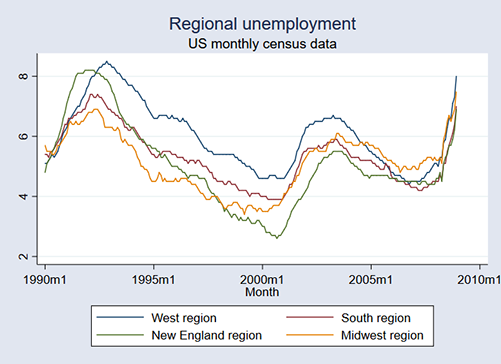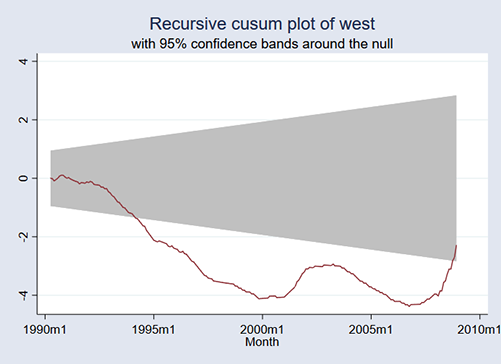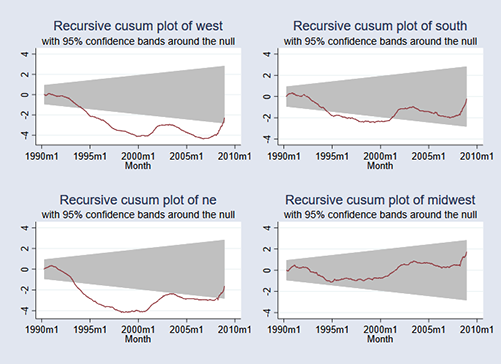»  Home »  Products »  Stata 15 »  Cumulative sum test for parameter stability

# Cumulative sum test for parameter stability

## Highlights

• Cumulative sum test for parameter stability
• Cumulative sum of recursive residuals
• Cumulative sum of OLS residuals
• Plot of cumulative sum of residuals with user-specified confidence plot

When you fit a time-series regression, you are assuming that the coefficients are stable over time. estat sbcusum tests that assumption. It bases its result on whether the time-series abruptly changes in ways not predicted by your model. Said more technically, it tests for structural breaks in the residuals.

estat sbcusum uses the cumulative sum of recursive residuals or the cumulative sum of OLS residuals to determine to test whether there is a structural break. Under the null hypothesis, the cumulative sum of residuals will have mean zero.

The command also graphs the cumulative sum with confidence bands, which allows you to see whether the series behaves as the null hypothesis would predict.

## Let's see it work

We have a simple model for unemployment. The model is that unemployment is a function of its lagged value. After we fit our model, we test for a structural break.

We have four series of unemployment, one for each Census region of the U.S. Those regions are West, South, New England, and Midwest. Variables west, south, ne, and midwest contain the corresponding unemployment. Our data are monthly and run from January 1990 to December 2008.

Here is a graph of the four series. Each of the series appears to have four turning points. They also appear to move together, but we will ignore that for the purpose of this example.Perhaps that will be tracked by our model. Let's begin with the Western region. We will fit our model by typing

. regress west L.west
(output omitted)


We can now perform the test for a structural break:

. estat sbcusum, name(west)

Cumulative sum test for parameter stability

Sample:  1990m2 - 2008m12                          Number of obs =        227
Ho: No structural break

1% Critical       5% Critical      10% Critical
Statistic   Test Statistic        Value             Value             Value

1% Critical       5% Critical      10% Critical
Statistic   Test Statistic        Value             Value             Value

recursive      2.0281            1.1430            0.9479             0.850



These results reject the null hypothesis of no structural break. Our simple model does not track the data. estat sbcusum also produced a graph:The red line is the cumulative sum. If the red line is the shaded area, the test would have not rejected the null hypothesis at the 5 percent level. The graph for the West is seldom in the shaded area!

We repeated this for the other four regions. Here are the four graphs put together:It appears that our model tracks the Midwestern region just fine, but it does not track the other three regions.

We chose to show you an example using four series and used sbcusum after fitting a simple model for each. When you use the command, you will probably be using it with one series and a more complicated, richer model that, you hope, will not reject the null hypothesis.